6.4.3 Step 3: Rebalance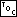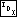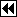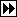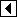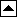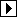The code in step 2 that inserts a node always colors the new node red. This means that rule 2 is always satisfied afterward (as long as it was satisfied before we began). On the other hand, rule 1 is broken if the newly inserted node's parent was red. In this latter case we must rearrange or recolor the BST so that it is again an RB tree.

This is what rebalancing does. At each step in rebalancing, we have the invariant that we just colored a node p red and that p's parent, the node at the top of the stack, is also red, a rule 1 violation. The rebalancing step may either clear up the violation entirely, without introducing any other violations, in which case we are done, or, if that is not possible, it reduces the violation to a similar violation of rule 1 higher up in the tree, in which case we go around again.

In no case can we allow the rebalancing step to introduce a rule 2 violation, because the loop is not prepared to repair that kind of problem: it does not fit the invariant. If we allowed rule 2 violations to be introduced, we would have to write additional code to recognize and repair those violations. This extra code would be a waste of space, because we can do just fine without it. (Incidentally, there is nothing magical about using a rule 1 violation as our rebalancing invariant. We could use a rule 2 violation as our invariant instead, and in fact we will later write an alternate implementation that does that, in order to show how it would be done.)

Here is the rebalancing loop. At each rebalancing step, it checks that we have a rule 1 violation by checking the color of pa[k - 1], the node on the top of the stack, and then divides into two cases, one for rebalancing an insertion in pa[k - 1]'s left subtree and a symmetric case for the right subtree. After rebalancing it recolors the root of the tree black just in case the loop changed it to red:

```201. <Step 3: Rebalance after RB insertion 201> =
while (k >= 3 && pa[k - 1]->rb_color == RB_RED)   {
if (da[k - 2] == 0)
{         <Left-side rebalancing after RB insertion 202>       }
else       {         <Right-side rebalancing after RB insertion 206>       }
}
tree->rb_root->rb_color = RB_BLACK;
```

This code is included in 197.

Now for the real work. We'll look at the left-side insertion case only. Consider the node that was just recolored red in the last rebalancing step, or if this is the first rebalancing step, the newly inserted node n. The code does not name this node, but we will refer to it here as q. We know that q is red and, because the loop condition was met, that its parent pa[k - 1] is red. Therefore, due to rule 1, q's grandparent, pa[k - 2], must be black. After this, we have three cases, distinguished by the following code:

```202. <Left-side rebalancing after RB insertion 202> =
struct rb_node *y = pa[k - 2]->rb_link;
if (y != NULL && y->rb_color == RB_RED)
{     <Case 1 in left-side RB insertion rebalancing 203>   }
else   {
struct rb_node *x;

if (da[k - 1] == 0)
y = pa[k - 1];
else       {         <Case 3 in left-side RB insertion rebalancing 205>       }

<Case 2 in left-side RB insertion rebalancing 204>
break;
}
```

This code is included in 201.

##### Case 1: q's uncle is red

If q has an “uncle” y, that is, its grandparent has a child on the side opposite q, and y is red, then rearranging the tree's color scheme is all that needs to be done, like this: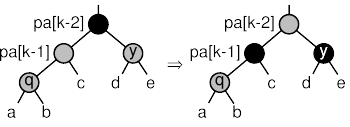Notice the neat way that this preserves the black-height (see black-height), or the number of black nodes in any simple path from a given node down to a node with 0 or 1 children, at pa[k - 2]. This ensures that rule 2 is not violated.

After the transformation, if node pa[k - 2]'s parent exists and is red, then we have to move up the tree and try again. The while loop condition takes care of this test, so adjusting the stack is all that has to be done in this code segment:

```203. <Case 1 in left-side RB insertion rebalancing 203> =
pa[k - 1]->rb_color = y->rb_color = RB_BLACK;
pa[k - 2]->rb_color = RB_RED;
k -= 2;
```

This code is included in 202, 207, 342, and 462.

##### Case 2: q is the left child of pa[k - 1]

If q is the left child of its parent, then we can perform a right rotation at q's grandparent, which we'll call x, and recolor a couple of nodes. Then we're all done, because we've satisfied both rules. Here's a diagram of what's happened: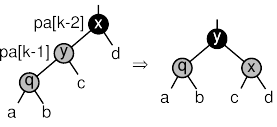There's no need to progress farther up the tree, because neither the subtree's black-height nor its root's color have changed. Here's the corresponding code. Bear in mind that the break statement is in the enclosing code segment:

```204. <Case 2 in left-side RB insertion rebalancing 204> =
x = pa[k - 2];
x->rb_color = RB_RED;
y->rb_color = RB_BLACK;

pa[k - 3]->rb_link[da[k - 3]] = y;
```

This code is included in 202, 343, and 464.

##### Case 3: q is the right child of pa[k - 1]

The final case, where q is a right child, is really just a small variant of case 2, so we can handle it by transforming it into case 2 and sharing code for that case. To transform case 2 to case 3, we just rotate left at q's parent, which is then treated as q.

The diagram below shows the transformation from case 3 into case 2. After this transformation, x is relabeled q and y's parent is labeled x, then rebalancing continues as shown in the diagram for case 2, with the exception that pa[k - 1] is not updated to correspond to y as shown in that diagram. That's okay because variable y has already been set to point to the proper node.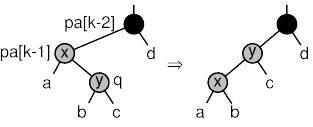```205. <Case 3 in left-side RB insertion rebalancing 205> =
x = pa[k - 1];